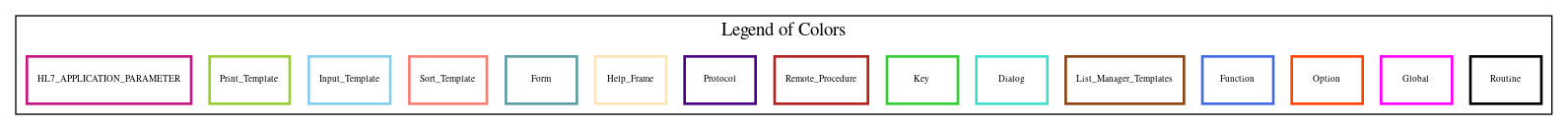Home   Package List   Routine Alphabetical List   Global Alphabetical List   FileMan Files List   FileMan Sub-Files List   Package Component Lists   Package-Namespace Mapping
Routine: LRVRMI4

# Routine: LRVRMI4

## Information

LRVRMI4 ;DALOI/STAFF - LAH/TMP TO FILE 63 ;09/07/16 08:09

## Source Information

Source file <LRVRMI4.m>

## Call Graph### Call Graph Total: 10

Package Total Call Graph
Lab Service 8 LEDI^LRMIAUD  VT1^LRMIUT1  SETPL^LRRPLUA  SETREF^LRUCSR  (N10,N13,N18,N7)^LRVRMI2  (N11,N12,N17,N9)^LRVRMI2A  (\$\$DUPCHK,NODE)^LRVRMI3  (BLDSTAT,N3,N6,SETSTAT)^LRVRMI4A
Kernel 1 \$\$NOW^XLFDT
VA FileMan 1 (FILE,UPDATE)^DIE

## Caller Graph

### Legends:#### Package Component Superscript legend

 action A extended action Ea event driver Ed subscriber Su protocol O limited protocol LP run routine RR broker B edit E server Se print P screenman SM inquire I### Caller Graph Total: 2

Package Total Caller Graph
Lab Service 2 LRVR0  LRVRMI4A

## Entry Points

EN ;
FIN ; Release report
N2 ; Process gram stain comments
N4 ; Bact report remarks
N99 ; Comment on specimen
AUDTRG ; Trigger the audit trail
CSR(LRCSR,LRFDAIEN,LRREF) ; Store code system references
; Call with LRCSR = array of ien/codes to store as references (pass by value)
; LRFDAIEN = FileMan array of entries added by DBA call (pass by value)
; LRREF = root of reference to build full reference to data

## External References

Name Field # of Occurrence
FILE^DIE EN+46, FIN+6, N2+31, N4+32, N99+8
UPDATE^DIE N2+18, N4+17
LEDI^LRMIAUD AUDTRG+7
VT1^LRMIUT1 EN+37
SETPL^LRRPLUA N2+22, N4+21, N99+10
SETREF^LRUCSR CSR+13
N10^LRVRMI2 EN+20
N13^LRVRMI2 EN+23
N18^LRVRMI2 EN+26
N7^LRVRMI2 EN+18
N11^LRVRMI2A EN+21
N12^LRVRMI2A EN+22
N17^LRVRMI2A EN+25
N9^LRVRMI2A EN+19
\$\$DUPCHK^LRVRMI3 N2+8, N4+10
NODE^LRVRMI3 EN+28
BLDSTAT^LRVRMI4A N2+15, N4+3
N3^LRVRMI4A EN+15
N6^LRVRMI4A EN+17
SETSTAT^LRVRMI4A EN+34
\$\$NOW^XLFDT EN+3

## Global Variables Directly Accessed

Name Line Occurrences  (* Changed,  ! Killed)
^LR - [#63] EN+6, EN+7, EN+10, N2+3, N4+5, N99+5
^TMP("LRMI" EN+2, EN+14, EN+15, EN+16, EN+17, EN+18, EN+19, EN+20, EN+21, EN+22
EN+23, EN+25, EN+26, EN+28, EN+30, N2+6, N2+7, N2+11, N4+2, N4+8
N4+9, N4+30, N99+2, N99+9

## Label References

Name Line Occurrences
AUDTRG EN+12, EN+42
CSR N2+25, N4+24
FIN EN+35
N2 EN+14
N4 EN+16
N99 EN+30

## Local Variables

### Legend:

 >> Not killed explicitly * Changed ! Killed ~ Newed

Name Field # of Occurrence
DIERR EN+43~, FIN+1~, N2+1~, N2+27!, N4+1~, N4+26!
DUZ FIN+3, N2+30, N4+31
I EN+1~, EN+10*, EN+11
IEN N2+1~, N2+5*, N2+6*, N2+7, N2+9, N2+11, N2+12, N2+13, N2+14, N2+20*
N2+21*, N2+22, N4+1~, N4+7*, N4+8*, N4+9, N4+11, N4+13, N4+14, N4+20*
N4+21*, CSR+5~, CSR+9*, CSR+10, CSR+11, CSR+12
LR63539 EN+1~, EN+5*, EN+7*, EN+42, EN+44
LRBATCH AUDTRG+1~, AUDTRG+2*
LRCMT N2+1~, N2+3*, N2+8, N4+1~, N4+5*, N4+10
LRCSR N2+1~, N2+25, N4+1~, N4+24, CSR~
LRCSR( N2+13*, N2+14*, N4+13*, N4+14*, CSR+12
LRDATA N2+1~, N99+1~, N99+2*, N99+3, N99+5, N99+7, AUDTRG+1~, AUDTRG+7, CSR+5~, CSR+13
LRDATA( AUDTRG+3*, AUDTRG+4*, AUDTRG+5*, AUDTRG+6*, CSR+12*
LRDATAREF CSR+5~, CSR+11*, CSR+12, CSR+13
LRDFN EN+2, EN+6, EN+7, EN+10, EN+14, EN+15, EN+16, EN+17, EN+18, EN+19
EN+20, EN+21, EN+22, EN+23, EN+25, EN+26, EN+28, EN+30, EN+44, FIN+2
N2+3, N2+6, N2+7, N2+9, N2+11, N2+22, N2+25, N2+28, N4+2, N4+5
N4+8, N4+9, N4+11, N4+21, N4+24, N4+27, N4+30, N99+2, N99+5, N99+6
N99+9, N99+10, CSR+5~, CSR+7*, CSR+13
>> LRDUZ FIN+3, N2+30, N4+31
LRFDA EN+43~, FIN+1~, N2+1~, N2+16, N2+27!, N4+1~, N4+15, N4+26!, N99+1~
LRFDA(1 EN+45*, FIN+3*, FIN+4*
LRFDA(2 N2+10*, N2+29*, N2+30*
LRFDA(4 N4+12*, N4+29*, N4+30*, N4+31*
LRFDA(99 N99+7*
LRFDAIEN N2+1~, N2+25, N4+1~, N4+24, CSR~
LRFDAIEN( N2+22, N4+21, CSR+10, CSR+11
>> LRIDT EN+2, EN+6, EN+7, EN+10, EN+14, EN+15, EN+16, EN+17, EN+18, EN+19
EN+20, EN+21, EN+22, EN+23, EN+25, EN+26, EN+28, EN+30, EN+44, FIN+2
N2+3, N2+6, N2+7, N2+9, N2+11, N2+22, N2+25, N2+28, N4+2, N4+5
N4+8, N4+9, N4+11, N4+21, N4+24, N4+27, N4+30, N99+2, N99+5, N99+6
N99+9, N99+10
LRIEN EN+43~, EN+44*, EN+45, FIN+1~, FIN+2*, FIN+3, FIN+4, N2+1~, N2+9*, N2+10
N2+28*, N2+29, N2+30, N4+1~, N4+11*, N4+12, N4+27*, N4+29, N4+30, N4+31
N99+6*, N99+7
LRIENS N2+1~, N2+27!, N4+1~, N4+26!
>> LRINTYPE EN+33, EN+41, N2+29, N4+28, AUDTRG+5, AUDTRG+6
>> LRLL N2+8, N4+10
LRMODE AUDTRG+1~, AUDTRG+2*
LRMSG EN+43~, FIN+1~, N2+1~, N2+27!, N4+1~, N4+26!, N99+1~
LRNODE EN+1~, EN+28*
LRNOW EN+1~, EN+3*, FIN+4, N2+29, N4+29, AUDTRG+3
LRPL N2+1~, N4+1~, N4+4*, N4+21
LRPL( N2+12*, N2+21, N2+22
>> LRPROF N2+8, N4+10
LRREF CSR~, CSR+7, CSR+11
LRROOT CSR+5~, CSR+7*, CSR+8*, CSR+9
LRRPTAPP EN+1~, EN+37, N2+32*, N4+33*
LRSB EN+11~*, EN+12
LRSTATUS EN+1~, EN+34, N2+15, N4+3
LRSTATUS(0 EN+35, EN+41, AUDTRG+4
LRX N2+1~, N2+7*, N2+8, N2+10, N2+11*, N2+12, N2+13, N2+14, N2+15, N4+1~
N4+2*, N4+3, N4+4, N4+9*, N4+10, N4+12, N4+13, N4+14, N99+1~, N99+9*
N99+10
ROLE CSR+5~, CSR+9*, CSR+12
STAT N2+1~, N2+5*
TYPE CSR+5~, CSR+9*, CSR+12
U N4+30
X EN+1~, EN+6*, EN+7